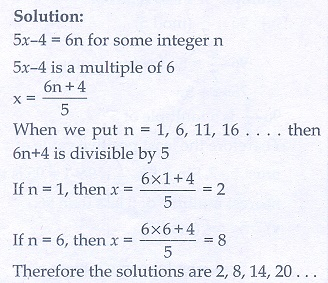Home | | Maths 10th Std | Exercise 2.3: Modular Arithmetic

# Exercise 2.3: Modular Arithmetic

Maths Book back answers and solution for Exercise questions - Mathematics : Numbers and Sequences: Modular Arithmetic: Exercise Problem Questions with Answer

Exercise 2.3

1. Find the least positive value of such that

(i) 71 ≡ x  (mod 8)

(ii) 78 + ≡ 3 (mod 5)

(iii) 89 ≡ (+ 3) (mod 4)

(iv) 96 ≡ x/7(mod 5)

(v) 5≡ 4(mod 6)2. If is congruent to 13 modulo 17 then 7- 3 is congruent to which number modulo 17?3. Solve 5≡ 4 (mod 6)4. Solve 3− 2 ≡ 0 (mod 11)5. What is the time 100 hours after 7 a.m.?6. What is the time 15 hours before 11 p.m.?7. Today is Tuesday. My uncle will come after 45 days. In which day my uncle will be coming?8. Prove that 2n + 6 × 9n is always divisible by 7 for any positive integer n.9. Find the remainder when 281 is divided by 17.10. The duration of flight travel from Chennai to London through British Airlines is approximately 11 hours. The airplane begins its journey on Sunday at 23:30 hours. If the time at Chennai is four and half hours ahead to that of London’s time, then find the time at London, when will the flight lands at London Airport.1.(i) 7 (ii) 5 (iii) 2 (iv) 7 (v) 2

2. 3

3. 2,8,14,…

4. 8, 19, 30, …

5. 11 a.m

6. 8 p.m

7. Friday

9. 2

10. 6 am, Monday

Tags : Problem Questions with Answer, Solution | Mathematics , 10th Mathematics : UNIT 2 : Numbers and Sequences
Study Material, Lecturing Notes, Assignment, Reference, Wiki description explanation, brief detail
10th Mathematics : UNIT 2 : Numbers and Sequences : Exercise 2.3: Modular Arithmetic | Problem Questions with Answer, Solution | Mathematics